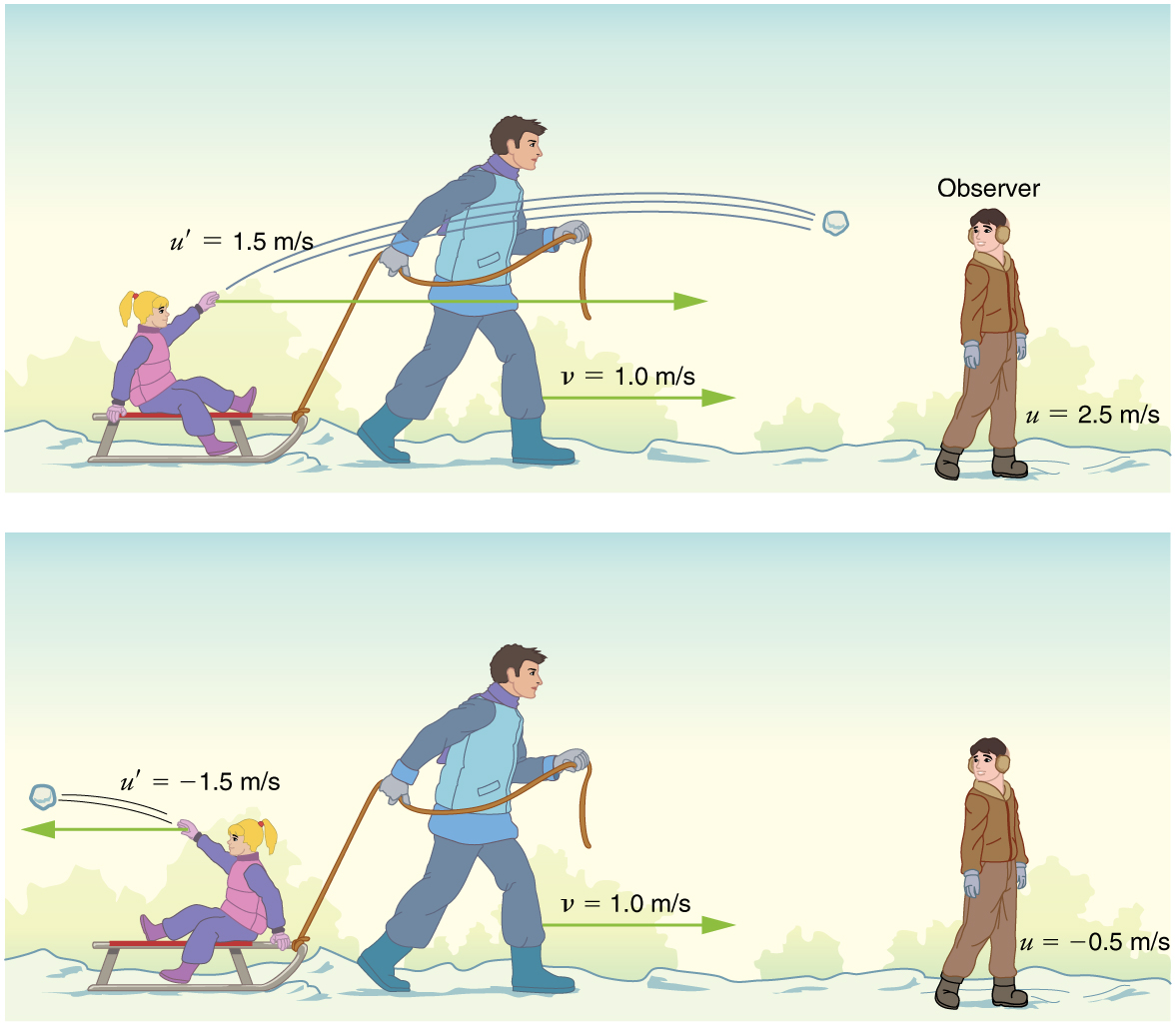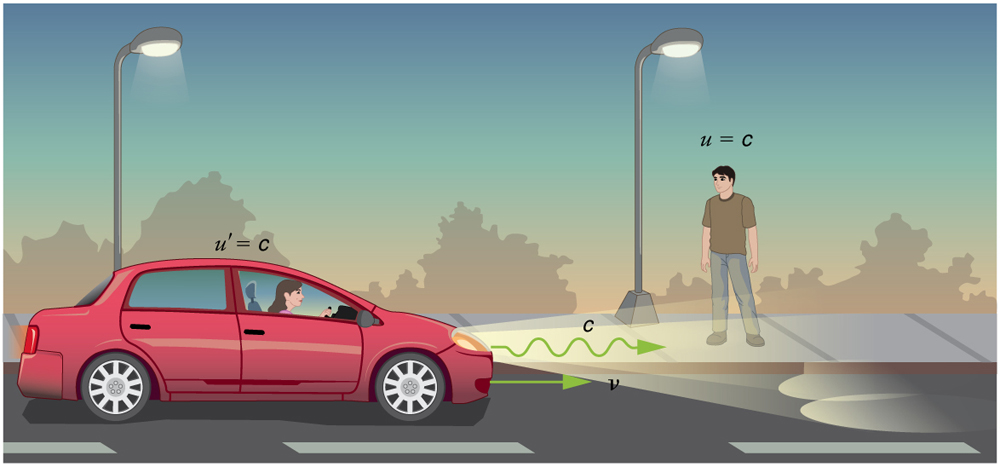# 28.4 Relativistic addition of velocities

 Page 1 / 7
• Calculate relativistic Doppler shift.The total velocity of a kayak, like this one on the Deerfield River in Massachusetts, is its velocity relative to the water as well as the water’s velocity relative to the riverbank. (credit: abkfenris, Flickr)

If you’ve ever seen a kayak move down a fast-moving river, you know that remaining in the same place would be hard. The river current pulls the kayak along. Pushing the oars back against the water can move the kayak forward in the water, but that only accounts for part of the velocity. The kayak’s motion is an example of classical addition of velocities. In classical physics, velocities add as vectors. The kayak’s velocity is the vector sum of its velocity relative to the water and the water’s velocity relative to the riverbank.

For simplicity, we restrict our consideration of velocity addition to one-dimensional motion. Classically, velocities add like regular numbers in one-dimensional motion. (See [link] .) Suppose, for example, a girl is riding in a sled at a speed 1.0 m/s relative to an observer. She throws a snowball first forward, then backward at a speed of 1.5 m/s relative to the sled. We denote direction with plus and minus signs in one dimension; in this example, forward is positive. Let $v$ be the velocity of the sled relative to the Earth, $u$ the velocity of the snowball relative to the Earth-bound observer, and $u\prime$ the velocity of the snowball relative to the sled.Classically, velocities add like ordinary numbers in one-dimensional motion. Here the girl throws a snowball forward and then backward from a sled. The velocity of the sled relative to the Earth is v= 1 . 0 m/s size 12{ ital "v="1 "." 0`"m/s"} {} . The velocity of the snowball relative to the truck is u ′ size 12{u rSup { size 8{'} } } {} , while its velocity relative to the Earth is u size 12{u} {} . Classically, u=v+u ′ .

$\text{u=v+u}\prime$

Thus, when the girl throws the snowball forward, $u=1.0 m/s+1.5 m/s=2.5 m/s$ . It makes good intuitive sense that the snowball will head towards the Earth-bound observer faster, because it is thrown forward from a moving vehicle. When the girl throws the snowball backward, $u=1.0 m/s+\left(-1.5 m/s\right)=-0.5 m/s$ . The minus sign means the snowball moves away from the Earth-bound observer.

The second postulate of relativity (verified by extensive experimental observation) says that classical velocity addition does not apply to light. Imagine a car traveling at night along a straight road, as in [link] . If classical velocity addition applied to light, then the light from the car’s headlights would approach the observer on the sidewalk at a speed $\text{u=v+c}$ . But we know that light will move away from the car at speed $c$ relative to the driver of the car, and light will move towards the observer on the sidewalk at speed $c$ , too.According to experiment and the second postulate of relativity, light from the car’s headlights moves away from the car at speed c size 12{c} {} and towards the observer on the sidewalk at speed c size 12{c} {} . Classical velocity addition is not valid.

What is the difference between a principle and a law
the law is universally proved. The principal depends on certain conditions.
Dr
it states that mass of an element deposited during electrolysis is directly proportional to the quantity of electricity discharge
Olamide
what does the speedometer of a car measure ?
Car speedometer measures the rate of change of distance per unit time.
Moses
describe how a Michelson interferometer can be used to measure the index of refraction of a gas (including air)
using the law of reflection explain how powder takes the shine off a person's nose. what is the name of the optical effect?
WILLIAM
is higher resolution of microscope using red or blue light?.explain
WILLIAM
what is dimensional consistent
Mohammed
In engineering and science, dimensional analysis is the analysis of the relationships between different physical quantities by identifying their base quantities and units of measure and tracking these dimensions as calculations or comparisons are performed
syed
can sound wave in air be polarized?
Unlike transverse waves such as electromagnetic waves, longitudinal waves such as sound waves cannot be polarized. ... Since sound waves vibrate along their direction of propagation, they cannot be polarized
Astronomy
A proton moves at 7.50×107m/s perpendicular to a magnetic field. The field causes the proton to travel in a circular path of radius 0.800 m. What is the field strength?
derived dimenionsal formula
what is the difference between mass and weight
assume that a boy was born when his father was eighteen years.if the boy is thirteen years old now, how is his father in
Isru
31yrs
Olamide
what is airflow
derivative of first differential equation
why static friction is greater than Kinetic friction
draw magnetic field pattern for two wire carrying current in the same direction
An American traveler in New Zealand carries a transformer to convert New Zealand’s standard 240 V to 120 V so that she can use some small appliances on her trip.
What is the ratio of turns in the primary and secondary coils of her transformer?
nkombo
what is energy
Yusuf
How electric lines and equipotential surface are mutually perpendicular?
The potential difference between any two points on the surface is zero that implies È.Ŕ=0, Where R is the distance between two different points &E= Electric field intensity. From which we have cos þ =0, where þ is the angle between the directions of field and distance line, as E andR are zero. Thus
sorry..E and R are non zero...

#### Get Jobilize Job Search Mobile App in your pocket Now!ByByBy Richley CrapoBy OpenStaxBy Keyaira BraxtonBy Angela JanuaryBy Edgar DelgadoBy Jonathan LongBy Melinda SalzerBy Mistry BhaveshBy OpenStaxBy John Gabrieli